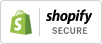# B7G MINIATURE BATTERY VALVES, THEIR EQUIVALENTS

Posted by STEVE MYCIUNKA on

A number of customers are now showing interest in B7G miniature battery valves so I thought I would do today’s blog on these marvelous little devices.  These first came to the fore in the early 1940s when they were utilized in personal radios particularly Stateside where they did duty in the likes of the Sonora Candid and the RCA BPA 10. As is usual, we didn’t catch up this side of the pond until the immediate postwar period with such designs as the Marconiphone P17B and Ever Ready Personal B. Let’s also not forget how these devices also saw duty throughout WW2 in a variety of man pack radios for the military.

The first B7G devices were produced by Sarnoff’s RCA and commercially distributed during 1939 and the line up comprised of the 1R5 – Heptode Frequency Changer; 1T4 – IF/RF amplifier; 1S5 – Detector Diode and AF amplifier all of these having 50mA 1.4V filaments but there was the; 1S4 Beam tetrode which had a 100mA filament and of course a version of the 1S4 with a 50mA + 50ma Centre tap filament capable of working as either a 3V 50mA series and 1.5V 100mA parallel. Mullard/Philips and Mazda/Adzam licensed copies of these contemporaneously and in 1953 launched their take which was the DK96. DF96, DAF96 and DL96 with improved construction which meant these had 25mA 1.4V filaments except the DL96 which is 50mA, or 25mA + 25ma centre tap.

To help with equivalent identification, I have prepared the following convenient equivalents listing: -

Heptode Frequency Changer 1R5 = DK91 = VT-171 = X17 = 1C1 = 1L6 = CV782
Heptode Frequency Changer 1AB3 = DK96 = VT-171 = X25 = 1C3

Enhanced Frequency Changer 1AC6 = DK92 = 1AC6 = X18 = X20 = 1C2 = CV5172

RF/IF Amplifier IT4 = DF91 = W17 = 1K2 = 1F31
RF/IF Amplifier IAJ4 = DF96 = W25 = 1F1

VHF RF/IF Amplifier IAN5 = DF97

Detector Diode/AF Preamplifier 1S4 = DL91 = 1S4 = CV783
Detector Diode/AF Preamplifier 1AH5 = 1AF33 = ZD25 = 1FD1 = DAF96

Detector Diode/AF Preamplifier (CT) 1S4 = DL912= 3S4 = N17 = 2P2 = 1P10 = CV820 = VT-174

Beam Tetrode DL93 = 3A4 = 2P3 = CV807
Beam Tetrode DL94 = 3V4 = N19 = 1P11 = CV1633
Beam Tetrode DL95 = 3Q4 = N18 = CV818
Beam Tetrode DL96 = 3C4 = N25 = 1P1

With the above information, the B7G affectionado will be able to number cross reference and match devices from various manufacturers and hopefully achieve thermionic nirvana!